# Difference between revisions of "Ergodicity"

of a dynamical system

2010 Mathematics Subject Classification: Primary: 37A25 [MSN][ZBL]

A property considered in ergodic theory. Originally it was defined for a cascade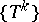or a flow (continuous-time dynamical system)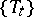with a finite invariant measurein the following way: If a function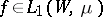is given on the phase space, then for almost-every point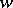the time average along the trajectory of this point exists, that is,orexists, and coincides with the space average (that is, with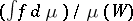). In this case one also speaks of ergodicity of. In particular, for any measurable set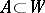the average time of a trajectory staying inis proportional to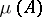for almost-every point (in fact, this property is equivalent to ergodicity). When the Birkhoff ergodic theorem had been proved it became clear that ergodicity is equivalent to metric transitivity. Therefore one spoke of ergodicity, meaning metric transitivity, in the more general situation when it was no longer suitable to talk of the equality of time and space averages (systems with an infinite invariant or quasi-invariant measure, not only flows and cascades, but also more general transformation groups and semi-groups).

How to Cite This Entry:
Ergodicity. Encyclopedia of Mathematics. URL: http://encyclopediaofmath.org/index.php?title=Ergodicity&oldid=17465
This article was adapted from an original article by D.V. Anosov (originator), which appeared in Encyclopedia of Mathematics - ISBN 1402006098. See original article